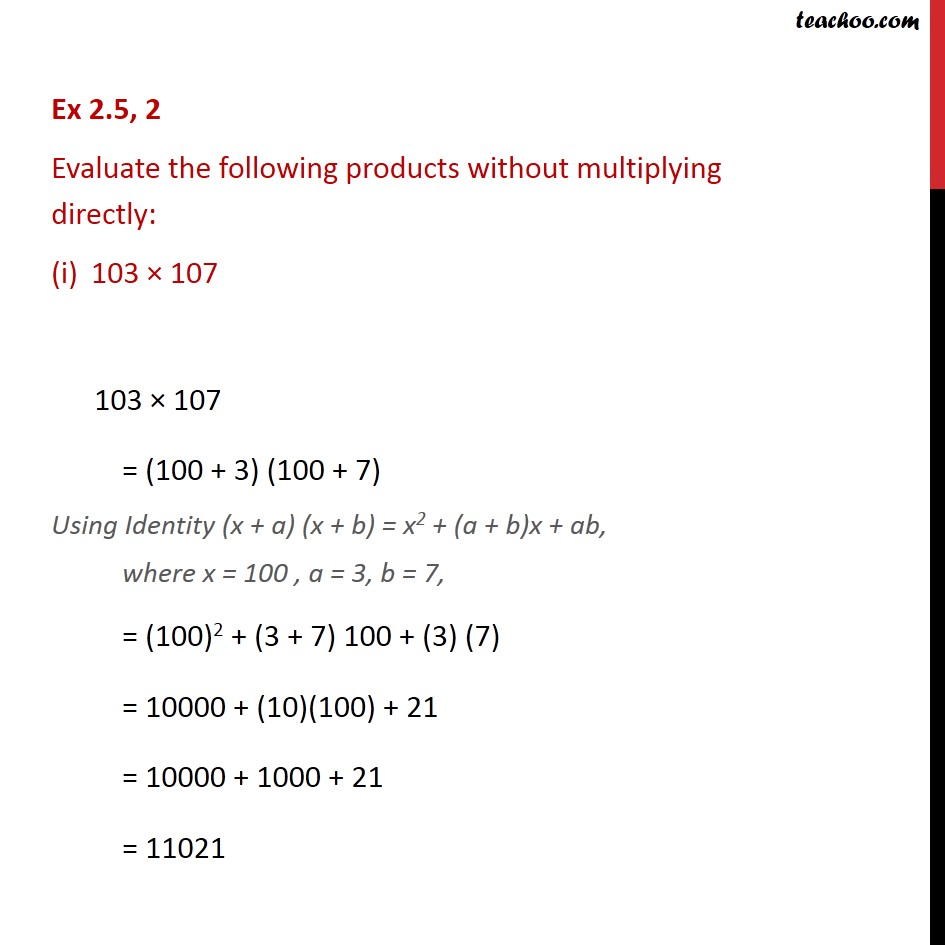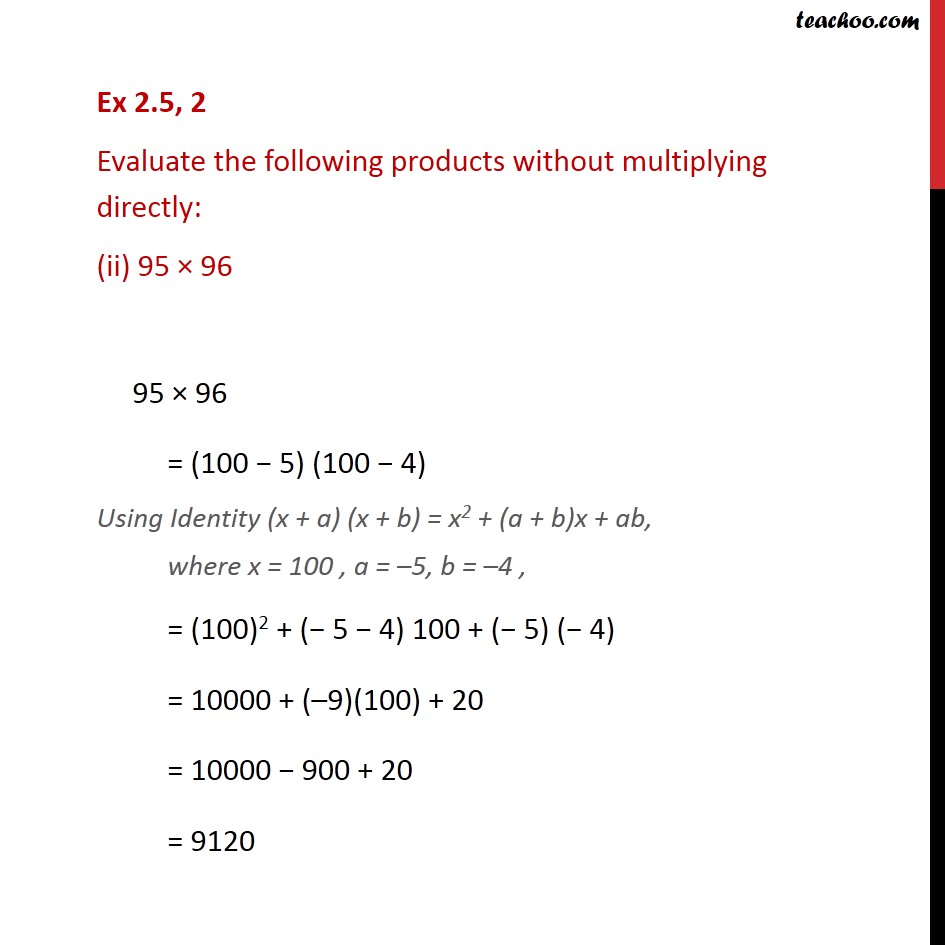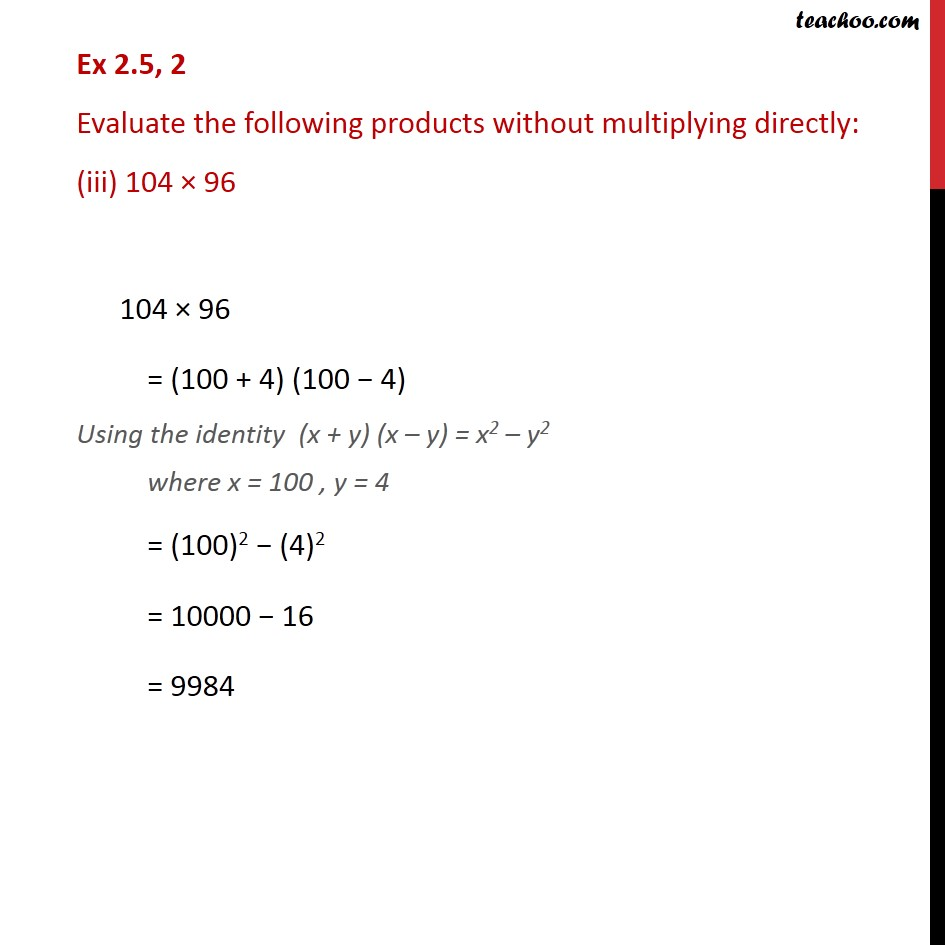1. Chapter 2 Class 9 Polynomials (Term 2)
2. Serial order wise
3. Ex 2.5

Transcript

Ex 2.5, 2 Evaluate the following products without multiplying directly: 103 107 103 107 = (100 + 3) (100 + 7) Using Identity (x + a) (x + b) = x2 + (a + b)x + ab, where x = 100 , a = 3, b = 7, = (100)2 + (3 + 7) 100 + (3) (7) = 10000 + (10)(100) + 21 = 10000 + 1000 + 21 = 11021 Ex 2.5, 2 Evaluate the following products without multiplying directly: (ii) 95 96 95 96 = (100 5) (100 4) Using Identity (x + a) (x + b) = x2 + (a + b)x + ab, where x = 100 , a = 5, b = 4 , = (100)2 + ( 5 4) 100 + ( 5) ( 4) = 10000 + ( 9)(100) + 20 = 10000 900 + 20 = 9120 Ex 2.5, 2 Evaluate the following products without multiplying directly: (iii) 104 96 104 96 = (100 + 4) (100 4) Using the identity (x + y) (x y) = x2 y2 where x = 100 , y = 4 = (100)2 (4)2 = 10000 16 = 9984

Ex 2.5